Inversesofof Trigonometric 10 4 Functions 10 4 Functions

• Slides: 27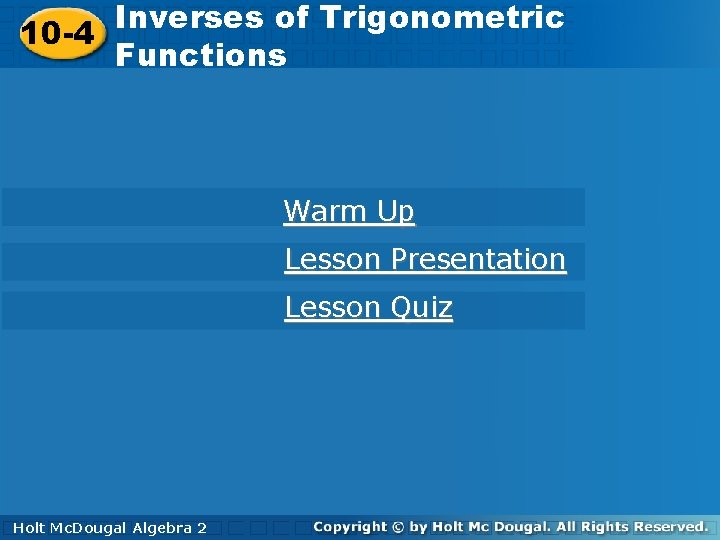Inversesofof. Trigonometric 10 -4 Functions 10 -4 Functions Warm Up Lesson Presentation Lesson Quiz Holt. Mc. Dougal Algebra 2 HoltInverses of Trigonometric 10 -4 Functions Warm Up Convert each measure from degrees to radians. 1. 120° 2. 180° 3. 225° 4. – 30° Holt Mc. Dougal Algebra 2Inverses of Trigonometric 10 -4 Functions Warm Up Find the exact value of each trigonometric function. 5. 6. 7. 8. Holt Mc. Dougal Algebra 2Inverses of Trigonometric 10 -4 Functions Objectives Evaluate inverse trigonometric functions. Use trigonometric equations and inverse trigonometric functions to solve problems. Holt Mc. Dougal Algebra 2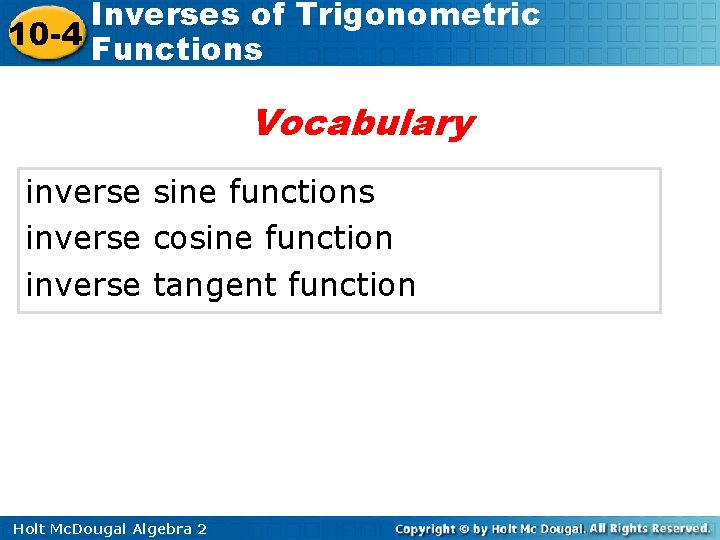Inverses of Trigonometric 10 -4 Functions Vocabulary inverse sine functions inverse cosine function inverse tangent function Holt Mc. Dougal Algebra 2Inverses of Trigonometric 10 -4 Functions You have evaluated trigonometric functions for a given angle. You can also find the measure of angles given the value of a trigonometric function by using an inverse trigonometric relation. Holt Mc. Dougal Algebra 2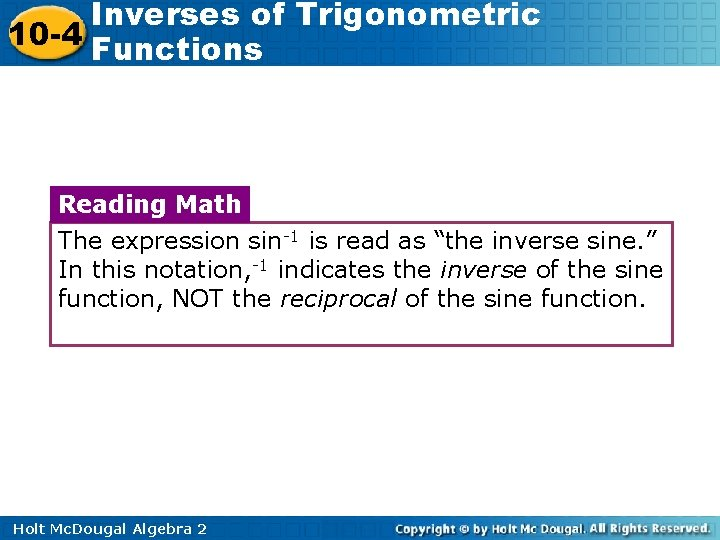Inverses of Trigonometric 10 -4 Functions Reading Math The expression sin-1 is read as “the inverse sine. ” In this notation, -1 indicates the inverse of the sine function, NOT the reciprocal of the sine function. Holt Mc. Dougal Algebra 2Inverses of Trigonometric 10 -4 Functions The inverses of the trigonometric functions are not functions themselves because there are many values of θ for a particular value of a. For example, suppose that you want to find cos-1 . Based on the unit circle, angles that measure and have a cosine of radians . So do all angles that are coterminal with these angles. Holt Mc. Dougal Algebra 2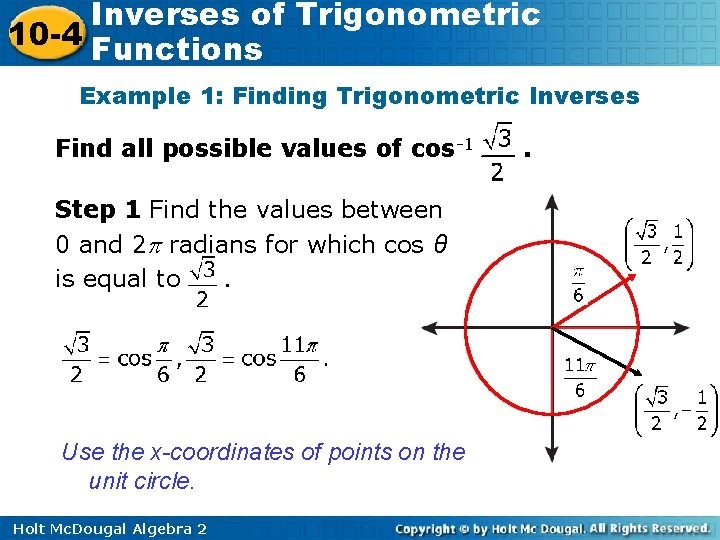Inverses of Trigonometric 10 -4 Functions Example 1: Finding Trigonometric Inverses Find all possible values of cos-1 Step 1 Find the values between 0 and 2 radians for which cos θ is equal to. Use the x-coordinates of points on the unit circle. Holt Mc. Dougal Algebra 2 .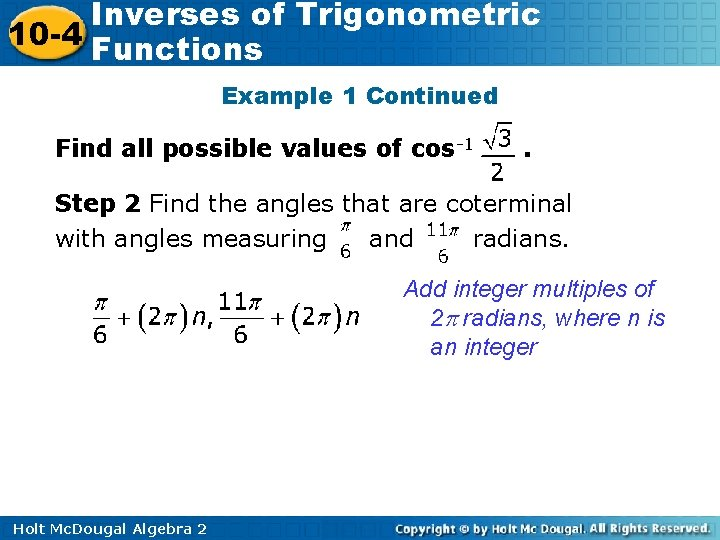Inverses of Trigonometric 10 -4 Functions Example 1 Continued Find all possible values of cos-1 . Step 2 Find the angles that are coterminal with angles measuring and radians. Add integer multiples of 2 radians, where n is an integer Holt Mc. Dougal Algebra 2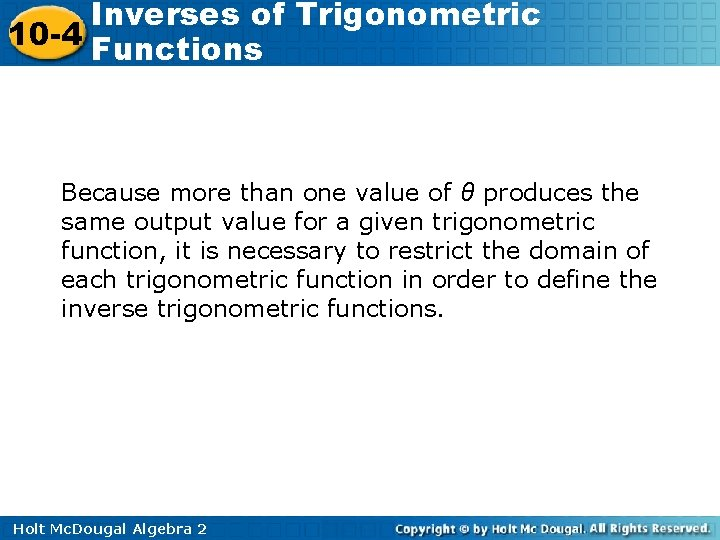Inverses of Trigonometric 10 -4 Functions Because more than one value of θ produces the same output value for a given trigonometric function, it is necessary to restrict the domain of each trigonometric function in order to define the inverse trigonometric functions. Holt Mc. Dougal Algebra 2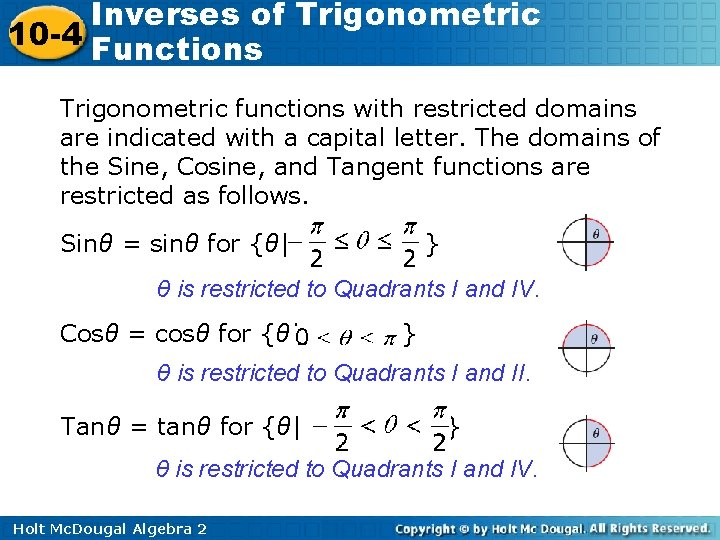Inverses of Trigonometric 10 -4 Functions Trigonometric functions with restricted domains are indicated with a capital letter. The domains of the Sine, Cosine, and Tangent functions are restricted as follows. Sinθ = sinθ for {θ| } θ is restricted to Quadrants I and IV. Cosθ = cosθ for {θ| } θ is restricted to Quadrants I and II. Tanθ = tanθ for {θ| } θ is restricted to Quadrants I and IV. Holt Mc. Dougal Algebra 2Inverses of Trigonometric 10 -4 Functions These functions can be used to define the inverse trigonometric functions. For each value of a in the domain of the inverse trigonometric functions, there is only one value of θ. Therefore, even though tan-1 has many values, Tan-11 has only one value. Holt Mc. Dougal Algebra 2Inverses of Trigonometric 10 -4 Functions Reading Math The inverse trigonometric functions are also called the arcsine, arccosine, and arctangent functions. Holt Mc. Dougal Algebra 2Inverses of Trigonometric 10 -4 Functions Example 2 A: Evaluating Inverse Trigonometric Functions Evaluate each inverse trigonometric function. Give your answer in both radians and degrees. Find value of θ for or whose Cosine. Use x-coordinates of points on the unit circle. Holt Mc. Dougal Algebra 2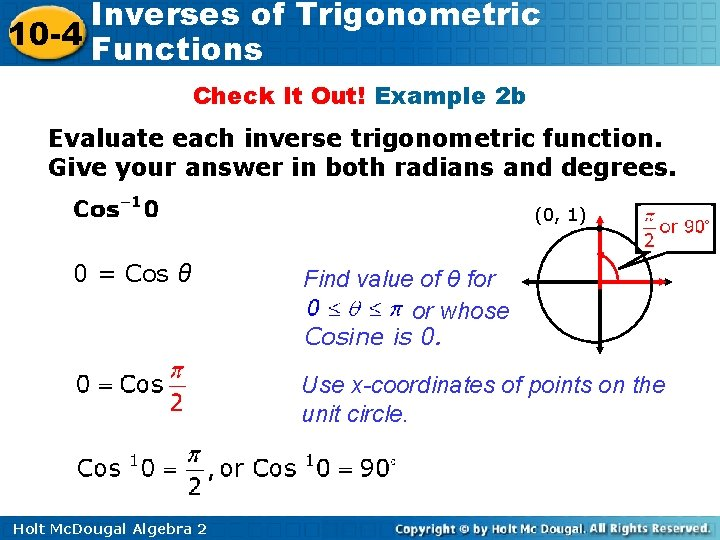Inverses of Trigonometric 10 -4 Functions Check It Out! Example 2 b Evaluate each inverse trigonometric function. Give your answer in both radians and degrees. (0, 1) 0 = Cos θ Find value of θ for or whose Cosine is 0. Use x-coordinates of points on the unit circle. Holt Mc. Dougal Algebra 2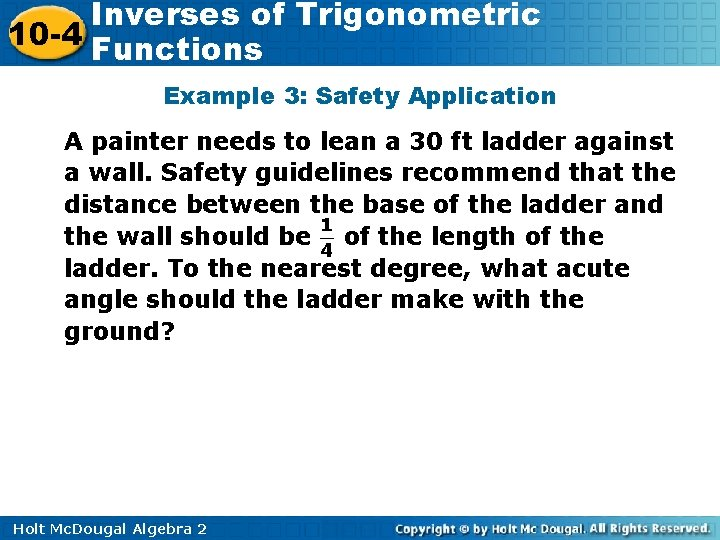Inverses of Trigonometric 10 -4 Functions Example 3: Safety Application A painter needs to lean a 30 ft ladder against a wall. Safety guidelines recommend that the distance between the base of the ladder and the wall should be of the length of the ladder. To the nearest degree, what acute angle should the ladder make with the ground? Holt Mc. Dougal Algebra 2Inverses of Trigonometric 10 -4 Functions Example 3 Continued Step 1 Draw a diagram. The base of the ladder should be (30) = 7. 5 ft from the wall. The angle between the ladder and the ground θ is the measure of an acute angle of a right triangle. 7. 5 Holt Mc. Dougal Algebra 2 θ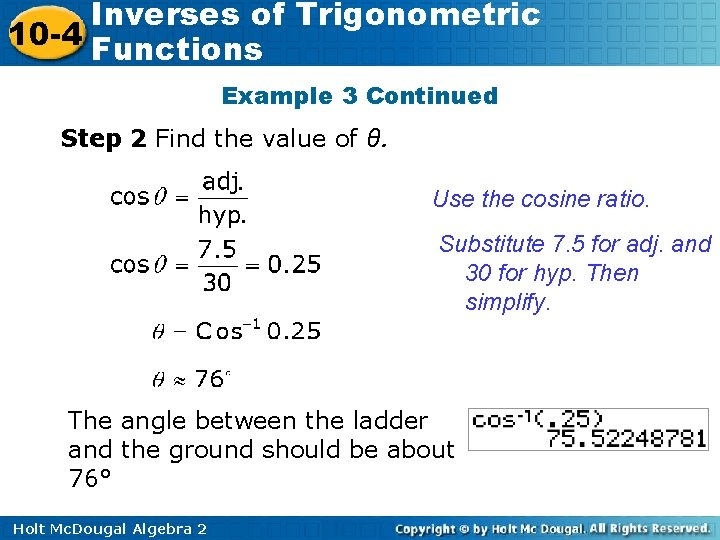Inverses of Trigonometric 10 -4 Functions Example 3 Continued Step 2 Find the value of θ. Use the cosine ratio. Substitute 7. 5 for adj. and 30 for hyp. Then simplify. The angle between the ladder and the ground should be about 76° Holt Mc. Dougal Algebra 2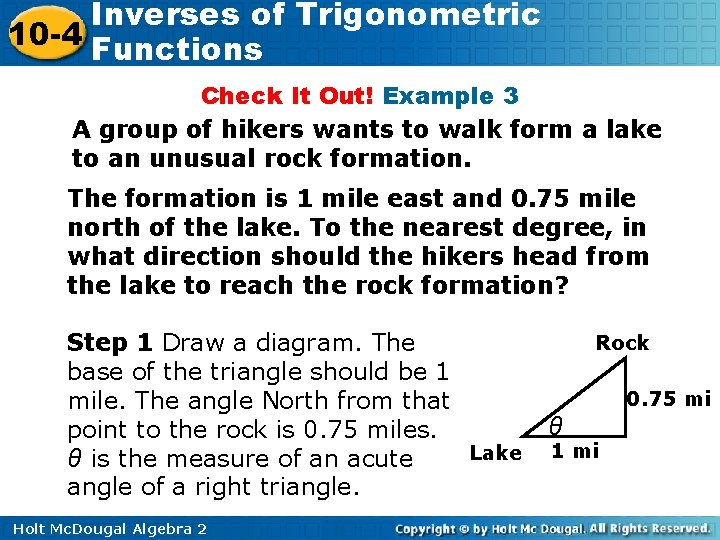Inverses of Trigonometric 10 -4 Functions Check It Out! Example 3 A group of hikers wants to walk form a lake to an unusual rock formation. The formation is 1 mile east and 0. 75 mile north of the lake. To the nearest degree, in what direction should the hikers head from the lake to reach the rock formation? Step 1 Draw a diagram. The base of the triangle should be 1 mile. The angle North from that point to the rock is 0. 75 miles. Lake θ is the measure of an acute angle of a right triangle. Holt Mc. Dougal Algebra 2 Rock 0. 75 mi θ 1 mi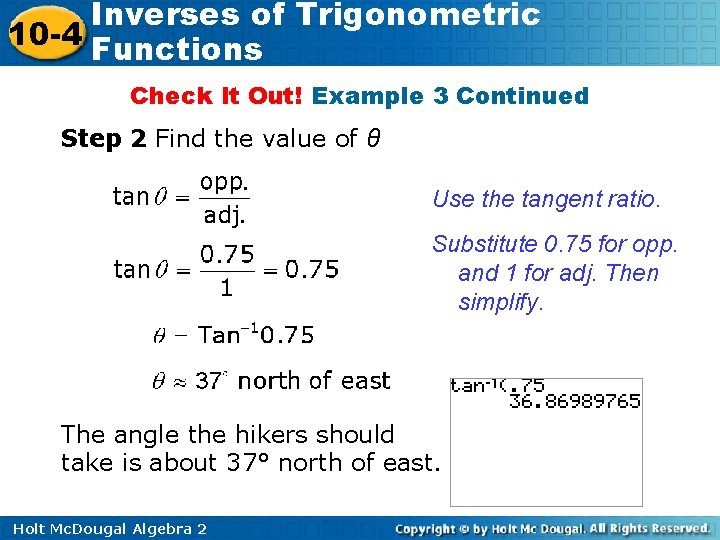Inverses of Trigonometric 10 -4 Functions Check It Out! Example 3 Continued Step 2 Find the value of θ Use the tangent ratio. Substitute 0. 75 for opp. and 1 for adj. Then simplify. The angle the hikers should take is about 37° north of east. Holt Mc. Dougal Algebra 2Inverses of Trigonometric 10 -4 Functions Example 4 A: Solving Trigonometric Equations Solve each equation to the nearest tenth. Use the given restrictions. sin θ = 0. 4, for – 90° ≤ θ ≤ 90° The restrictions on θ are the same as those for the inverse sine function. = Sin-1(0. 4) ≈ 23. 6° Holt Mc. Dougal Algebra 2 Use the inverse sine function on your calculator.Inverses of Trigonometric 10 -4 Functions Example 4 B: Solving Trigonometric Equations Solve each equation to the nearest tenth. Use the given restrictions. sin θ = 0. 4, for 90° ≤ θ ≤ 270° The terminal side of θ is restricted to Quadrants ll and lll. Since sin θ > 0, find the angle in Quadrant ll that has the same sine value as 23. 6°. θ has a reference angle of 23. 6°, and θ ≈ 180° – 23. 6° ≈ 156. 4° 90° < θ < 180°. Holt Mc. Dougal Algebra 2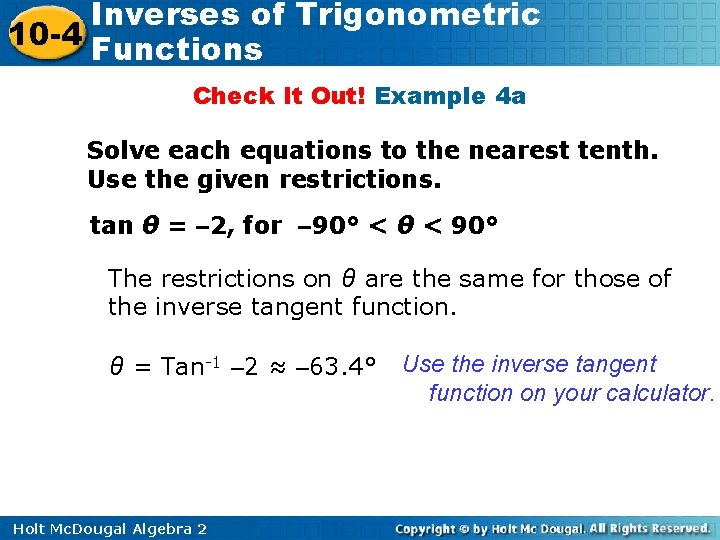Inverses of Trigonometric 10 -4 Functions Check It Out! Example 4 a Solve each equations to the nearest tenth. Use the given restrictions. tan θ = – 2, for – 90° < θ < 90° The restrictions on θ are the same for those of the inverse tangent function. θ = Tan-1 – 2 ≈ – 63. 4° Holt Mc. Dougal Algebra 2 Use the inverse tangent function on your calculator.Inverses of Trigonometric 10 -4 Functions Check It Out! Example 4 b Solve each equations to the nearest tenth. Use the given restrictions. tan θ = – 2, for 90° < θ < 180° The terminal side of θ is restricted to Quadrant II. Since tan θ < 0, find the angle in Quadrant II that has the same value as – 63. 4°. 116. 6° – 63. 4° θ ≈ 180° – 63. 4° ≈ 116. 6° Holt Mc. Dougal Algebra 2Inverses of Trigonometric 10 -4 Functions Lesson Quiz: Part I 1. Find all possible values of cos-1(– 1). 2. Evaluate Sin-1 and degrees. Give your answer in both radians 3. A road has a 5% grade, which means that there is a 5 ft rise for 100 ft of horizontal distance. At what angle does the road rise from the horizontal? Round to the nearest tenth of a degree. 2. 9° Holt Mc. Dougal Algebra 2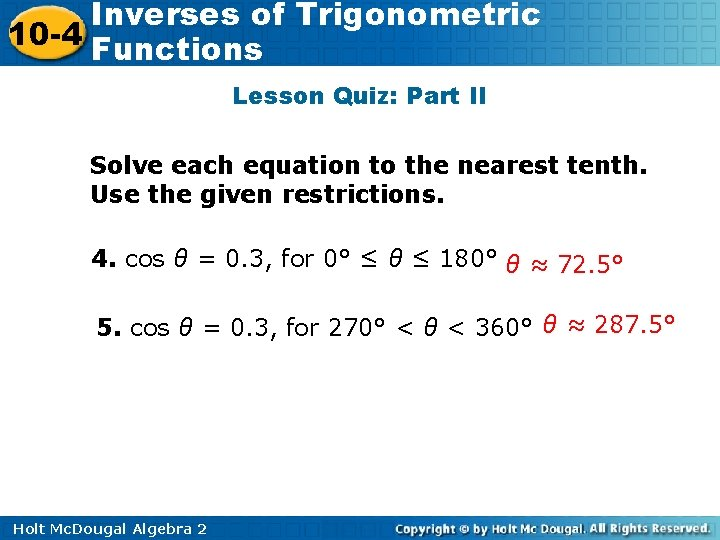Inverses of Trigonometric 10 -4 Functions Lesson Quiz: Part II Solve each equation to the nearest tenth. Use the given restrictions. 4. cos θ = 0. 3, for 0° ≤ θ ≤ 180° θ ≈ 72. 5° 5. cos θ = 0. 3, for 270° < θ < 360° θ ≈ 287. 5° Holt Mc. Dougal Algebra 2